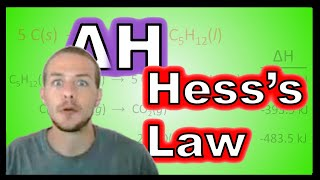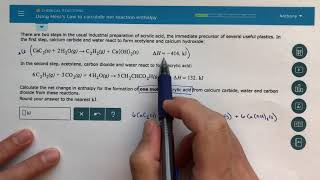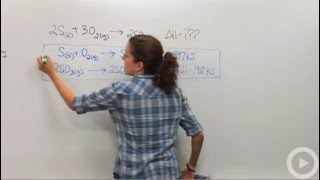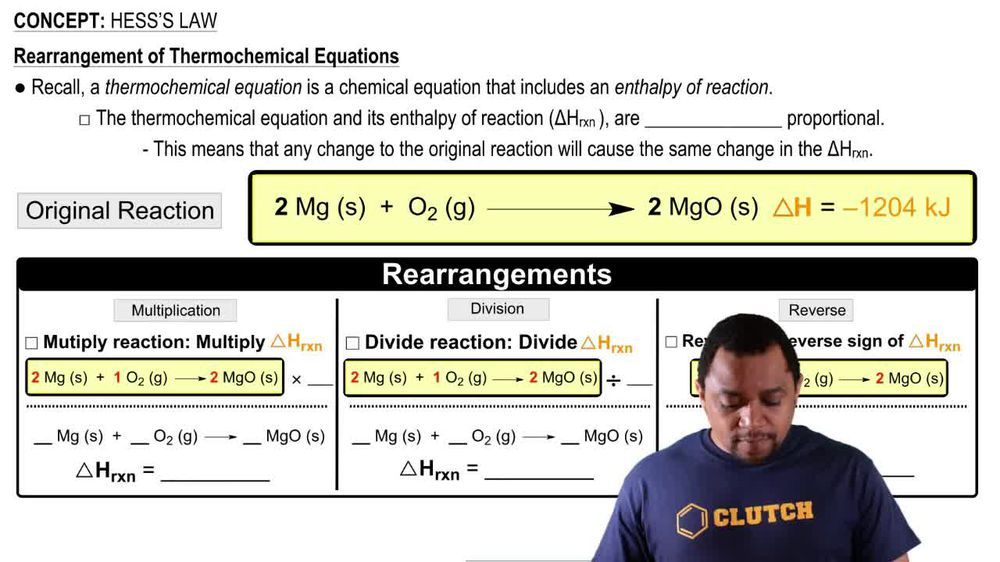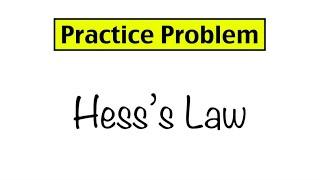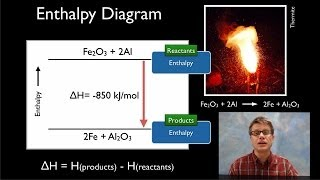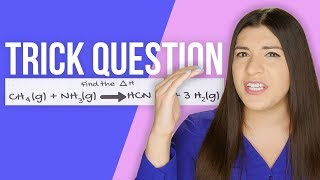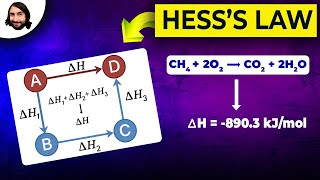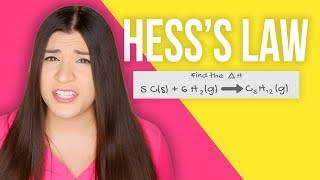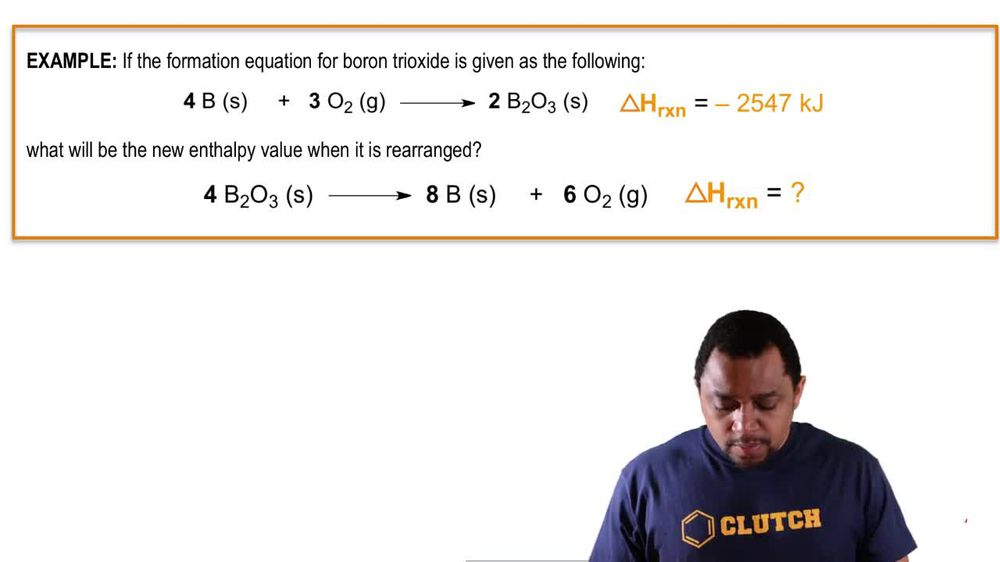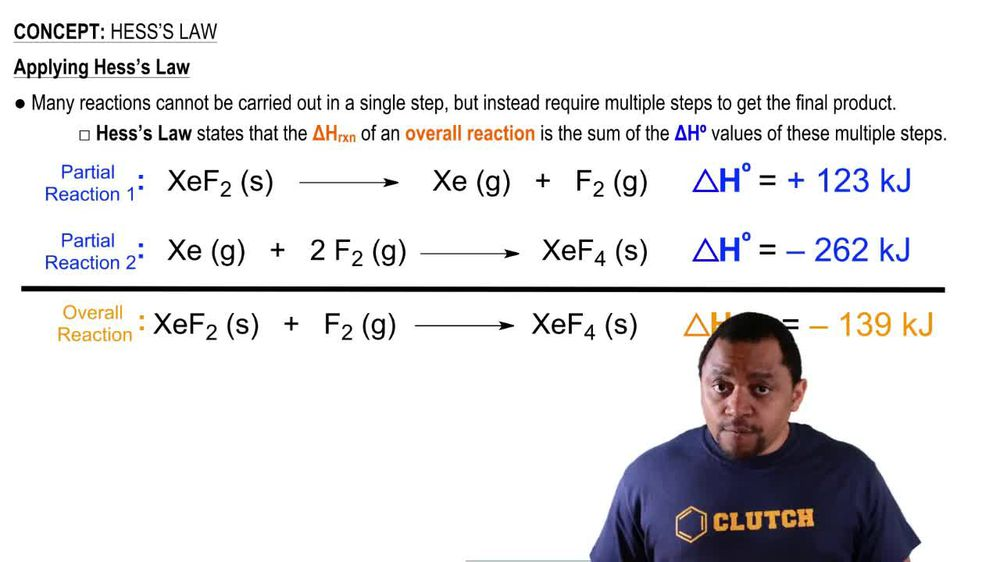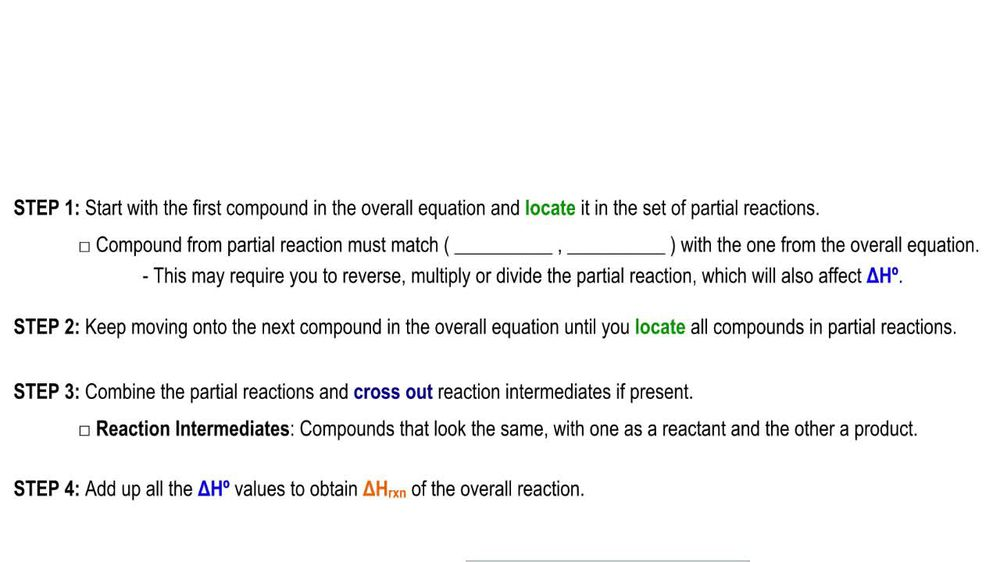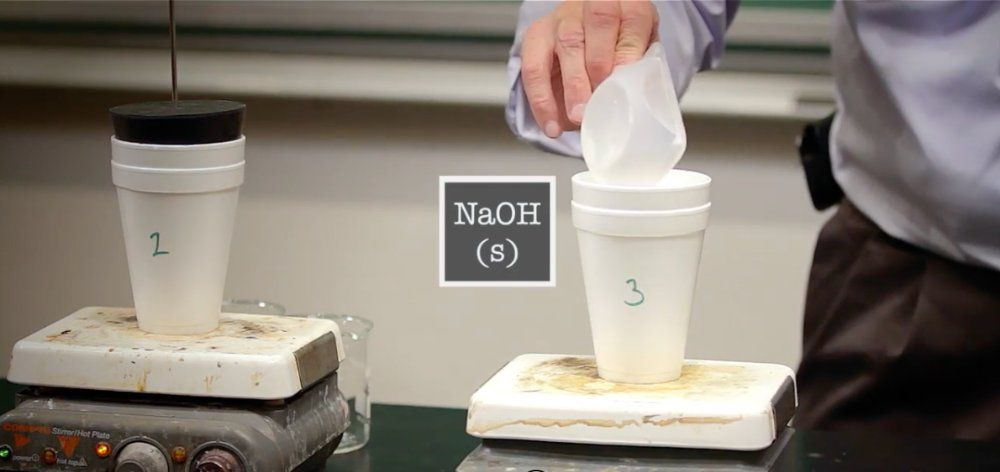Start typing, then use the up and down arrows to select an option from the list.
1. 8. Thermochemistry2. Hess's Law# Hess's Law Example 2

by Jules Bruno
139 views
1
1
1
here, it says to calculate the MPP of reaction for the overall reaction. So we have carbon monoxide plus nitrogen monoxide producing carbon dioxide and half a mole of nitrogen gas. Now here were given these partial reactions below with their own known standard mantelpiece off reaction. Now what do we do? Well, let's follow the rules. Step one says that I need to start with the first compound in the overall equation and located in the set of partial reactions. Compound from partial reaction must match in terms of number and type. We'll see what that means with one from the overall equation. This may require you to reverse, multiply or divide the partial reaction, which will also affect your standard entropy value. Step two says. Keep moving on to the next compound in the overall equation until locate all compounds in the partial reaction. So let's do steps one and two initially, all right, so we have to. So if we go back to step one, it says that we need to locate the compound. We must locate the first comment in the overall equation and located in the set off partial reactions. Alright, so in my overall equation. I have carbon monoxide gas as a reactant, and there's one of it, one mole of it. So if I look in the partial reactions, we see carbon monoxide is right here. But we have an issue. Although there is one mole of it like I want it is a product we needed to match up with the overall equation. We needed to be a reactant to do that. That means I'm gonna have to reverse the reaction. Now when I reverse the reaction, it's now gonna become our reactant. Oh, to also becomes a reactant and co two becomes a product. Now, remember, when I reverse the reaction, that's going to reverse the sign for Delta H. So now, my carbon monoxide, there's one mole of it. So the number is good and the type is also good because it's also reacted now, just like in my overall equation. This equation is gone now because it's been reversed and is represented by this bottom one. Next, I need to find no gas. I needed to be one mole of it, and I needed to be a reactant. Here I see it. Two issues First, it has a two in front of it. I needed to have a coefficient of one, just like in my overall equation. Also, I needed to be a reactant. So what I'm gonna do here is I'm going to divide by two and reverse. Okay, so here, I'm gonna divide by two and reverse it. So that's gonna become an out gas. And then when I reverse and when I divide by two, this becomes half end to okay. Plus half +02 when I reversed the equation. Yeah. Remember that's going to change. The science of this becomes negative. And when I divide by two, I have to divide Delta H by two. So this is negative. 90.3 killer jewels. So now this animal matches up exactly with the overall equation. Keep going. We need one mole of co two, his product, which we have here. We'd have a mole of and to as product, which we have here. So all the compounds I need match up with the overall equation. So now we go to Step three, combine the partial reactions and cross that reaction intermediates if present reaction intermediates or compounds that look the same with one as a reactant and the other as a product. Once we do that, we go to step forward. We add all the standard entropy, values off my partial reactions to obtain the entropy of reaction of the overall reaction. All right, so who are intermediates? Things that look the same but one's a product and one's reacted. We have half Oh, to here as a reactant which will cancel out with this half 02 That's a product. Those are Onley reaction intermediates. Everything else comes down. If you look when everything comes down, it should give you back the overall equation you are looking for in the first place. It doesn't matter. The order that you write them in All that matters is that the coefficients match the overall equation and that they're on the right side. Reactant is here. Should match up with the reactions up in the overall products here should match up with the products up here. All we do now is we add up these mental piece of reaction. So when I add up negative to 83 plus this negative 90.3, that gives me a total entropy off reaction equal to negative 3. 73.3 killer jewels. So this would be our Hess's law question where we solved for the overall entropy of the reaction for the overall reaction.